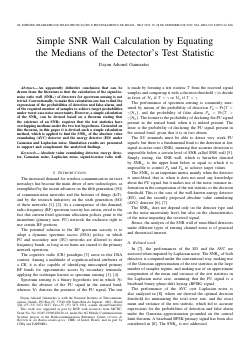##### Simple SNR Wall Calculation by Equating the Medians of the Detector's Test Statistic

###### Abstract
An apparently definitive conclusion that can be drawn from the literature is that the calculation of the signal-to-noise ratio wall (SNRw) of detectors for spectrum sensing is not trivial. Conventionally, to make this calculation one has to find the expressions of the probabilities of detection and false alarm, and of the required number of samples to achieve target probabilities under worst-case noise uncertainty. However, a simple calculation of the SNRw can be devised based on a theorem stating that the existence of an SNRw requires that the test statistics have overlapping medians under the two test hypotheses. Grounded on this theorem, in this paper it is devised such a simple calculation method, which is applied to find the SNRw of the absolute value cumulating (AVC) detector and the energy detector (ED) under Gaussian and Laplacian noise. Simulation results are presented to support and complement the analytical findings.